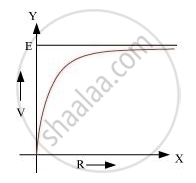# A Cell of Emf ‘E’ and Internal Resistance ‘R’ is Connected Across a Variable Resistor ‘R’. Plot a Graph Showing the Variation of Terminal Potential ‘V’ with Resistance R. Predict from the Graph - Physics

#### Question

A cell of emf ‘E’ and internal resistance ‘r’ is connected across a variable resistor ‘R’. Plot a graph showing the variation of terminal potential ‘V’ with resistance R. Predict from the graph the condition under which ‘V’ becomes equal to ‘E’.

#### Solution

V becomes equal to E when no current flows through the circuit.The condition under which V will be equal to E is when R = ∞

Is there an error in this question or solution?

#### Video TutorialsVIEW ALL 

A Cell of Emf ‘E’ and Internal Resistance ‘R’ is Connected Across a Variable Resistor ‘R’. Plot a Graph Showing the Variation of Terminal Potential ‘V’ with Resistance R. Predict from the Graph Concept: Cells, Emf, Internal Resistance.